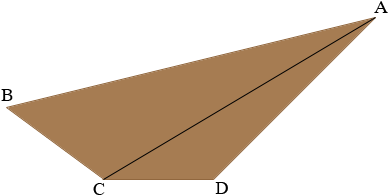SEARCH HOMEMath Central Quandaries & QueriesQuestion from Jean, a student: A railway cut 200ft. long and 30ft. wide at the bottom is made with sloping sides, which are 80ft. and 60ft. in length. The 80-ft. side is inclined 45 degrees and the other side is 35 degrees to the horizontal. Find the cost of removing the earth at \$2 per load, if the trucks have capacity of 4cu. yd.Jean,

The volume of the cut is the area of the cross-section times the length which is 200 feet. The cross-section is a quadrilateral.The area of the quadrilateral is the sum of the areas of the triangles ABC and ACD. If you can determine the lengths of the triangle sides you can find their areas using Heron's Formula.

You know the length of BC, CD and DA and the measures of the angles BCD and CDA. Use the Law of Cosines with the triangle ACD to find the length of AC. Use the Law of Sines with the same triangle to find the measure of the angle ACD.

Can you see how to proceed?

PennyMath Central is supported by the University of Regina and The Pacific Institute for the Mathematical Sciences.